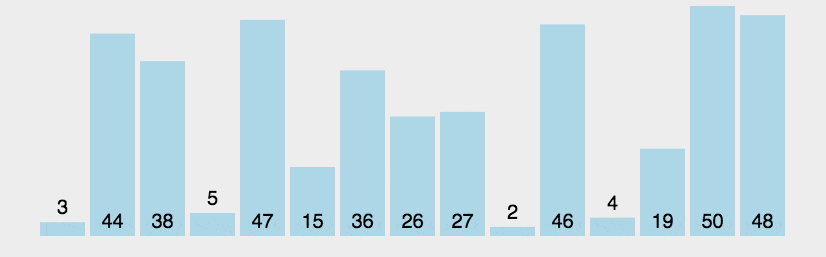# Java array bubble sorting and judging whether the array is orderly

Suunsr 2022-05-22 12:25:44 阅读数:992

javaarraybubblesortingjudging

# One . Bubble sort :

Bubble sort （Bubble Sort） It is also a simple and intuitive sorting algorithm . It repeatedly visits the sequence to be sorted , Compare two elements at a time , If they're in the wrong order, exchange them . The job of the interview sequence is to repeat until there is no need to exchange , That is to say, the sequence has been sorted . The name of this algorithm comes from the fact that the smaller the elements, the more slowly " floating " Go to the top of the list .

As one of the simplest sorting algorithms , Bubble sorting makes me feel like Abandon It feels the same in a word book , Every time on the first page , So most familiar with . Bubble sorting also has an optimization algorithm , It's about making a flag, When elements are not exchanged in a sequence traversal , It is proved that the sequence has been ordered . But this improvement doesn't do much to improve performance .

### 1. Algorithm steps

Compare adjacent elements . If the first one is bigger than the second one , Just swap them .

Do the same for each pair of adjacent elements , From the beginning of the first couple to the end of the last couple . After this step , The last element will be the maximum number .

Repeat the above steps for all elements , Except for the last one .

Keep repeating the above steps for fewer and fewer elements each time , Until there's no pair of numbers to compare .

2. Dynamic diagram demonstration``````public class Bubblesort Test {
public static void main(String[] args) {
int arr[] = new int[]{9,5,1,6,4,3,2,8,7};
bubbleSort(arr);
System.out.println(Arrays.toString(arr));// Output the array as characters
}
private static void bubbleSort(int[] arr) {
// The outer loop represents the number of times
for (int i = 0; i < arr.length-1; i++) {
boolean isSwaped = false;// Set up flag
for (int j = 0; j < arr.length-1-i; j++) {
// The memory loop is used to compare the size of the two
if(arr[j] > arr[j+1]){
// Ascending exchange
int tmp = arr[j];
arr[j] = arr[j+1];
arr[j+1] = tmp;
isSwaped = true;
}
}
if(!isSwaped){
break;
}
}
}
}``````

# Two . Is it orderly

``````public class Homework11_20 {
public static void main(String[] args) {
int arr[] = new int[]{1, 2, 3, 4, 5, 6};
int arr1[] = new int[]{2, 5, 1, 3, 6, 8};
boolean ret = isSorted(arr);
boolean ret1 = isSorted(arr1);
System.out.println(ret);
System.out.println(ret1);
}
private static boolean isSorted(int[] arr) {
for (int i = 0; i < arr.length - 1; i++) {
if (arr[i] > arr[i+1]){
return false;// Disordered output false
}
}
return true;// Ordered output true
}
}``````